Courses

# Test: Electrical Machines- 8

## 10 Questions MCQ Test GATE Electrical Engineering (EE) 2022 Mock Test Series | Test: Electrical Machines- 8

Description
This mock test of Test: Electrical Machines- 8 for Electrical Engineering (EE) helps you for every Electrical Engineering (EE) entrance exam. This contains 10 Multiple Choice Questions for Electrical Engineering (EE) Test: Electrical Machines- 8 (mcq) to study with solutions a complete question bank. The solved questions answers in this Test: Electrical Machines- 8 quiz give you a good mix of easy questions and tough questions. Electrical Engineering (EE) students definitely take this Test: Electrical Machines- 8 exercise for a better result in the exam. You can find other Test: Electrical Machines- 8 extra questions, long questions & short questions for Electrical Engineering (EE) on EduRev as well by searching above.
QUESTION: 1

### A 3-phase synchronous motor of 10 KW at 1.3 kV has a synchronous reactance of 10 Ω/phase. The efficiency of the machine is 75%. Find input power for the motor.

Solution:

Given that, output to the motor (Pout) = 10 kW
Efficiency (η) = 0.75
Motor line current is minimum at cos ϕ = 1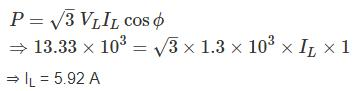Input to motor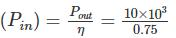= 13.33 kW

QUESTION: 2

### A synchronous motor with negligible armature resistance runs at a load angle of 20° at the rated frequency. If supply frequency is increased by 10%, keeping the other parameters constant, the new load angle will be

Solution:

Power delivered by the synchronous motor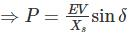From data, other parameters such as P, E and V are constant.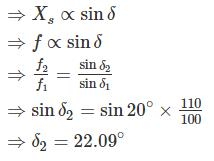*Answer can only contain numeric values
QUESTION: 3

### The per phase circuit equivalent of a synchronous generator feeding a synchronous motor through a transmission line is shown in fig. Each machine is rated 10 kVA, 400V, 50 Hz. The motor is driving a load of 8 kW. The field current of the two machine are so adjusted that the motor terminal voltage is 400V and its pf is 0.8 leading. Find the minimum value of Em (in P.U) for the machine to remain in synchronism.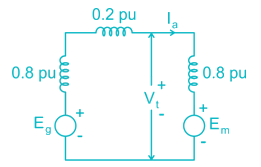Solution: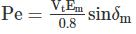For maximum Em, δm = 90° limit of stability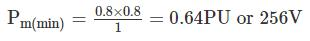QUESTION: 4

The rotor of a 50 Hz, 4 poles, 3ϕ synchronous motor is directly coupled to the rotor of a 50 Hz, 6 poles induction motor. If the stators of both machines are given balanced 3ϕ, 50 Hz supplies of rated voltage values. The possible induced frequencies in the rotor of induction motor are-

Solution:

We know that, N = 120f/P
Speed of synchronous motor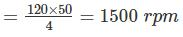Speed of induction motor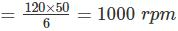Given that both the rotors are directly coupled.
If rotating field is in the opposite direction to that of rotor speed.
Speed = 1500 + 1000 = 2500 rpm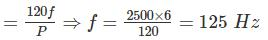If rotating field is in the same direction to that of rotor speed,
Speed = 1500 – 1000 = 500 rpm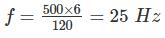*Answer can only contain numeric values
QUESTION: 5

A cylindrical rotor synchronous motor is used to improve the power factor of a load 5 MW at a power factor of 0.6 lag. The motor is required to meet an additional demand of 0.2 MW and raise the overall power factor to 0.8 (lag). If the efficiency of the motor is 75%. The power factor of the motor is – (up to two decimal places)

Solution:

Rating of load is –
P1 = 5 MW, cos ϕ1 = 0.6
Q1 = P1 tan ϕ
= 5 × tan (cos-1 (0.6)) = 6.67 MVAR
Required motor output = 0.2 MW
Efficiency = 0.75Total load = 5 + 0.267 = 5.267 MW
New power factor cos ϕ = 0.8 lag.
Reactive power, Q = P tan ϕ
= 5.267 × tan (cos-1 (0.8))
= 3.95 MVAR
MVA supplied by motor, Q2 = Q1 – Q = 6.67 – 3.95 = 2.72 MVAR
Q2 = P2 tan ϕ2
⇒ 2.72 = 0.267 tanϕ2
⇒ ϕ2 = 84.39°
Cosϕ2 = 0.098

*Answer can only contain numeric values
QUESTION: 6

A 60 MVA, 11 kV, 50 Hz, Y connected, 3 phase salient pole synchronous motor has direct axis synchronous reactance Xd = 1.8 Ω and quadrature axis synchronous reactance Xq = 1.2 Ω. If the armature resistance is negligible and full load power factor is 0.8 log. The power angle is-

Solution: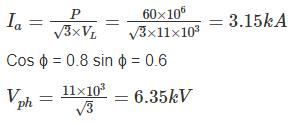For logging load in salient pole motor,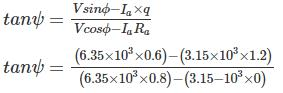ψ = 0.338°
δ + ψ = 3.338°
⇒ δ = 0.338° - 36.86° = -36.52°

*Answer can only contain numeric values
QUESTION: 7

A 3-phase synchronous motor of 10 KW at 1.3 kV has synchronous reactance of 10 Ω/phase. The efficiency of the machine is 75%. The induced emf (per phase) corresponding to minimum current for full load condition is ____ (in V). Neglect armature resistance.

Solution:

Given that, output to motor (Pout) = 10 kW
Efficiency (η) = 0.75
Motor line current is minimum at cos ϕ = 1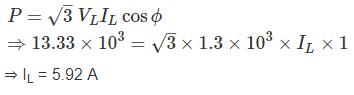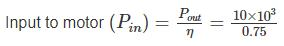= 13.33 kW
Impedance drop = I × synchronous impedance
= 5.92 × 10 = 59.20 V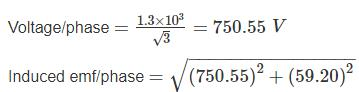= 752.89 V

*Answer can only contain numeric values
QUESTION: 8

A 400 V, 3ϕ delta connected synchronous motor runs at rated voltage and with an excitation emf of 500 V. Its winding and iron losses are 1800 W and synchronous impedance per phase is (0.7 + 3j) Ω. Calculate the shaft power output in kW

Solution:

Zs = (0.7 + 3j) = 3.08 ∠76.87
Vt = 400 V, Ef = 500 V
Maximum out put power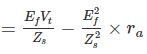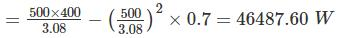Shaft power = [3 × 46.49 – 1.8]kW = 137.66 kW

*Answer can only contain numeric values
QUESTION: 9

The maximum power delivered by 1500 kW, three phase star connected 4 kV, 48 pole 50 Hz synchronous motor, with synchronous reactance of 4 Ω per phase and unity power factor is___(in MW)

Solution: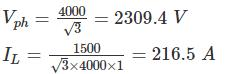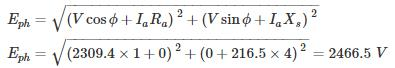QUESTION: 10

A 2300 V, 400 h.p, 60 Hz, 8 pole Y connected synchronous motor has a rated power factor of 0.85 leading. At full load, the efficiency 85%. The armature resistance is 0.4, and the synchronous reactance is 4.4 Ω. Find the Ea for this machine when it is operating at full load.

Solution: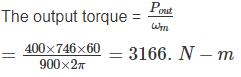The input power is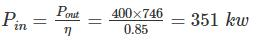The armature current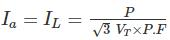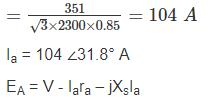= 1328 ∠0° - 0.4 × 104∠31.8° - j × 4.4 × 104∠31.8°

Ea = 1588 ∠-15° V